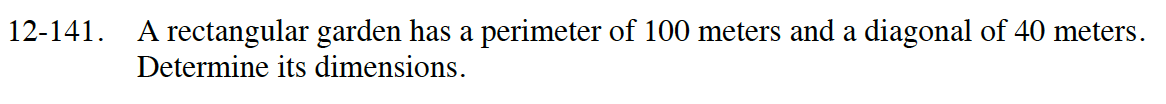### Home > INT2 > Chapter 12 > Lesson 12.2.4 > Problem12-141

12-141.

A rectangular garden has a perimeter of 100 meters and a diagonal of 40 meters. Determine its dimensions. Homework Help ✎Write the equation for the perimeter and solve for one of the variables.

Using the same variables, write the equation for a triangle formed with the diagonal.

Solve for the dimensions (variables) as you would a system of equations.

Length = 38.2 m
Width = 11.8 m